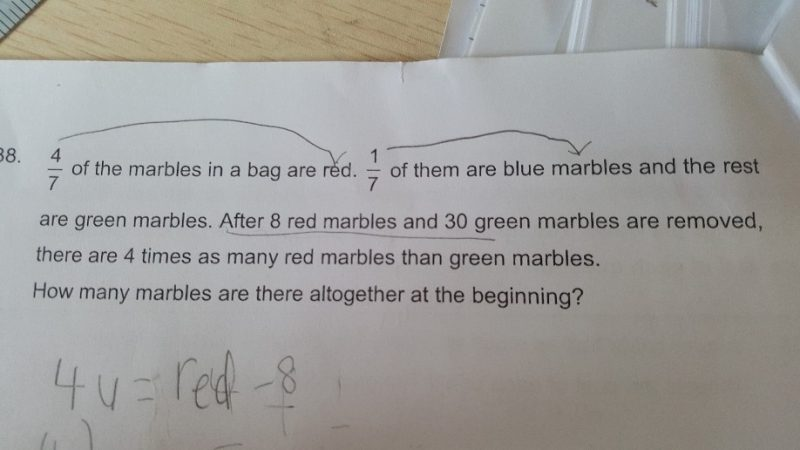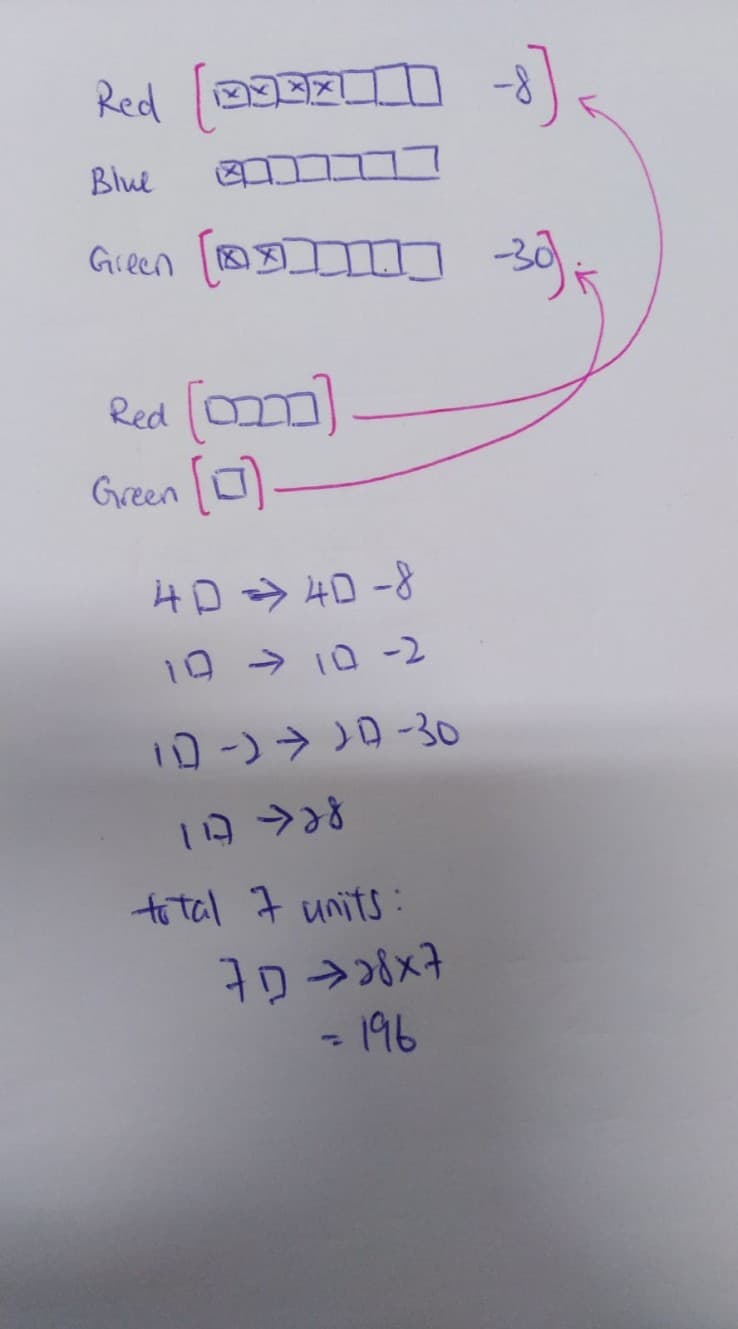# Question0 Replies 0 Likes

I’ll try to explain as clear as I can.

a) Total Red = 4u +8

b) Total Green = u + 30

c) Total Blue = 1/7

Given total red is 4/7, (a) can also be rewrite as:

4/7 = 4u+8

1/7 = (4u+8) / 4

=u + 2 (which is also Blue)

We know that Green is 2/7 and Blue is 1/7,

Green – Blue = 1/7

(u+30) – (u+2) = 1/7

1/7 = 28 Marbles

Total Marbles = 28 * 7

= 196 Marbles

*But I’m not sure if P4 is learning such method. If someone else can come out with a P4 method please share.

0 Replies 0 Likes